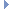OpenMM
BrownianIntegrator Class Reference

This is an Integrator which simulates a System using Brownian dynamics. More...Inheritance diagram for BrownianIntegrator:

List of all members.

## Public Member Functions

def getTemperature
getTemperature(self) -> double
def setTemperature
Set the temperature of the heat bath (in Kelvin).
def getFriction
getFriction(self) -> double
def setFriction
Set the friction coefficient which determines how strongly the system is coupled to the heat bath (in inverse ps).
def getRandomNumberSeed
getRandomNumberSeed(self) -> int
def setRandomNumberSeed
Set the random number seed.
def step
Advance a simulation through time by taking a series of time steps.
def __init__
__init__(self, temperature, frictionCoeff, stepSize) -> BrownianIntegrator __init__(self, other) -> BrownianIntegrator

this

## Detailed Description

This is an Integrator which simulates a System using Brownian dynamics.

## Constructor & Destructor Documentation

 def __init__ ( self, args )

__init__(self, temperature, frictionCoeff, stepSize) -> BrownianIntegrator __init__(self, other) -> BrownianIntegrator

Create a BrownianIntegrator.

Parameters:
 temperature (double) the temperature of the heat bath (in Kelvin) frictionCoeff (double) the friction coefficient which couples the system to the heat bath, measured in 1/ps stepSize (double) the step size with which to integrate the system (in picoseconds)

## Member Function Documentation

 def getFriction ( self )

getFriction(self) -> double

Get the friction coefficient which determines how strongly the system is coupled to the heat bath (in inverse ps).

Returns:
(double) the friction coefficient, measured in 1/ps
 def getRandomNumberSeed ( self )

getRandomNumberSeed(self) -> int

Get the random number seed. See setRandomNumberSeed() for details.

 def getTemperature ( self )

getTemperature(self) -> double

Get the temperature of the heat bath (in Kelvin).

Returns:
(double) the temperature of the heat bath (in Kelvin).
 def setFriction ( self, coeff )

Set the friction coefficient which determines how strongly the system is coupled to the heat bath (in inverse ps).

Parameters:
 coeff (double) the friction coefficient, measured in 1/ps
 def setRandomNumberSeed ( self, seed )

Set the random number seed.

The precise meaning of this parameter is undefined, and is left up to each Platform to interpret in an appropriate way. It is guaranteed that if two simulations are run with different random number seeds, the sequence of random forces will be different. On the other hand, no guarantees are made about the behavior of simulations that use the same seed. In particular, Platforms are permitted to use non-deterministic algorithms which produce different results on successive runs, even if those runs were initialized identically.

If seed is set to 0 (which is the default value assigned), a unique seed is chosen when a Context is created from this Force. This is done to ensure that each Context receives unique random seeds without you needing to set them explicitly.

 def setTemperature ( self, temp )

Set the temperature of the heat bath (in Kelvin).

Parameters:
 temp (double) the temperature of the heat bath, measured in Kelvin.
 def step ( self, steps )

Advance a simulation through time by taking a series of time steps.

Parameters:
 steps (int) the number of time steps to take

Reimplemented from Integrator.

## Member Data Documentation

Reimplemented from Integrator.

The documentation for this class was generated from the following file:
• openmm.py